JAVA, HTML5 & Chemistry Site

Other Writing

Last Update
19-Oct-2014

### Chemical Engineering: Activity coefficients estimation by ASOG (Analytical Solutions of Groups) method

2011.4.15

Lecture note of Dr. Hiroshi Yamamoto

## <!-- document.write("<p>Check the browser <BR>"); var userAgent = window.navigator.userAgent.toLowerCase(); var appVersion = window.navigator.appVersion.toLowerCase(); var canrun=0; if (userAgent.indexOf("msie") > -1) { if (appVersion.indexOf("msie 6.0") > -1) { document.write("IE 6.0 does not support HTML5. Please use Chrome, Safari or FireFox. <BR>"); } else if (appVersion.indexOf("msie 7.0") > -1) { document.write("IE 7.0 does not support HTML5. Please use Chrome, Safari or FireFox. <BR>"); } else if (appVersion.indexOf("msie 8.0") > -1) { document.write("IE 8.0 does not support HTML5. Please use Chrome, Safari or FireFox. <BR>"); } else if (appVersion.indexOf("msie 9.0") > -1) { document.write("IE9 may run this simulator but out of support. Please use Chrome, Safari or FireFox. <BR>"); } else { document.write("Unkown Browser. Please use Chrome, Safari or FireFox. <BR>"); } } else if (userAgent.indexOf("firefox") > -1) { canrun=1; } else if (userAgent.indexOf("opera") > -1) { canrun=1; } else if (userAgent.indexOf("chrome") > -1) { canrun=1; } else if (userAgent.indexOf("safari") > -1) { canrun=1; } else { document.write("Unkown Browser. Please use Chrome, Safari or FireFox. <BR>"); } if(canrun==1){ document.write("This Browser will run HTML5. <BR>"); } // -->

HTML5 version (2011.4.19) How to use
JAVA version (1999.2)

### Activity Coefficient:

Wilson equation and NRTL equation are very convenient activity coefficient calculating equation that introduce partial molar fraction. With this equations, we can estimate vapor liquid equilibrium (VLE) for multi components system with Wilson parameters that are determined from binary VLE experimental data. But if there are 1000 solvents, we need 1000*999 pair of Wilson parameters. It is impossible to get all of them.
So, Solutions of Groups model such as ASOG and UNIFAC were developed. The Solution of Groups method divid molecule into Functional Groups such as CH3, OH, O=C< and COOH, and the model assumed that the properties of the solution are determined by the interaction of Group pair.

I explain this model more detail.

At first, Activity Coefficients (see Wiki for more detail)
Activity Coefficients is something like concentration that fix the error between ideal solution and real solution. I will explain more easily.
Suppose,
there are 2 material A and B.
Xa and Xb are molar fractions in vapor phase.
Pa and Pb are vapor pressure.
.
Then we can write following schemes.

P(total pressure)= Pa + Pb
Pa=Xa*P
Pb=Xb*P
If Xa=1 (molar fraction of A in vapor phase = 1), then Xb should be 0, and Pa=P(total). In the vapor phase, there are only A component exist, so total pressure should be Pa. If the combination of A, B is ideal, the result of scanning liquid phase ratio 0-1 would become figure like below.

The total pressure become summation of each component’s pressure. This relationship is called Raoult law. But almost all real liquids are not play like this.

If Xa=1 (molar fraction of A in vapor phase = 1), then Xb should be 0, and Pa=P(total). In the vapor phase, there are only A component exist, so total pressure should be Pa. If the combination of A, B is ideal, the result of scanning liquid phase ratio 0-1 would become figure like below.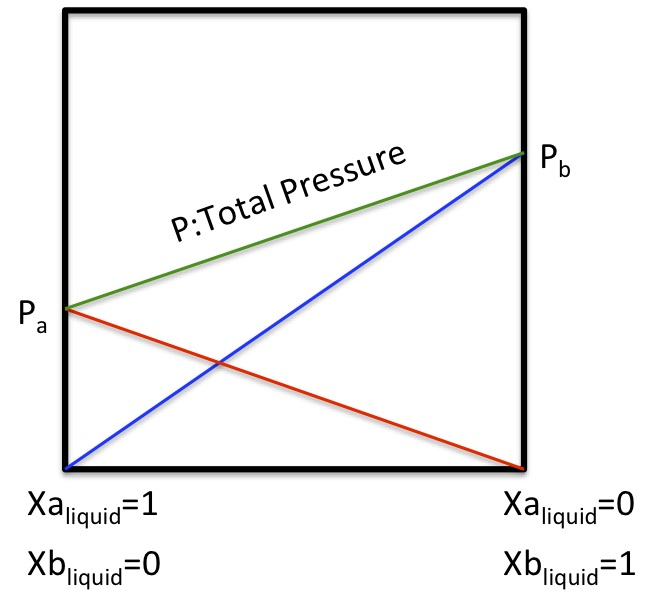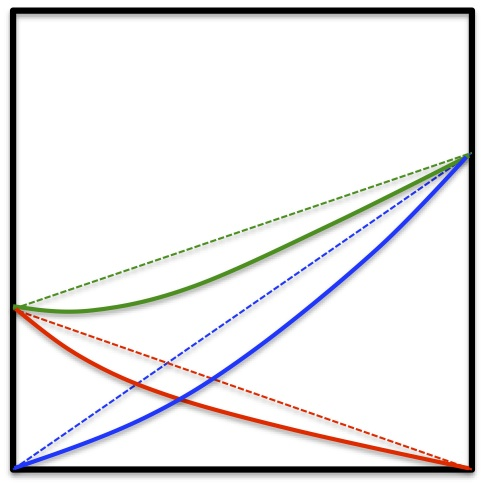sometime it goes negative.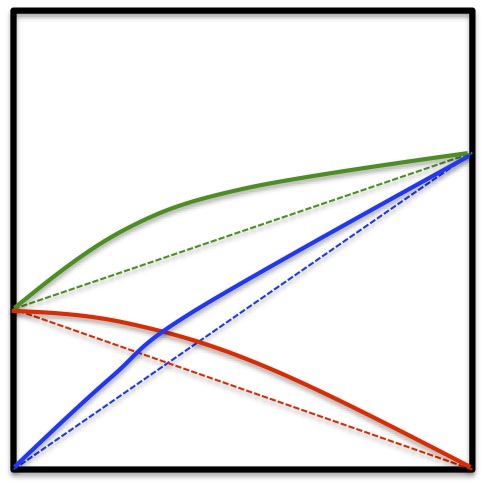sometime it goes positive.
The pair that pretend ideal, are Benzene-Toluene or Hexane-Heptane system.
The pair that show negative shift, are Acetone-Chloroform system.
For positive shift, Carbon tetrachloride- Methanol system.
With simple explanation, if the structures are similar, it play rather ideal. So activity coefficients are introduced by Gilbert Lewis for the pair that chemical structures are different.

P*yi = γi *xi *Psi
P: total pressure,
yi: molar fraction i in vapor phase.
xi: molar fraction i in liquid phase
Psi: vapor pressure of i component.
γi : Activity Coefficient of i component.

If we assume γi *xi as effective concentration, this equation back to the Raoult law (ideal solution).
For ideal pair like Benzene-Toluene or Hexane-Heptane system, γbecome 1.
What is the meaning of negative shift?
Some group pair interact strongly and hard to evaporate at that temperature. And positive shift means that some group pair do not want to stay same phase and put out. It leads more evaporation at that temperature.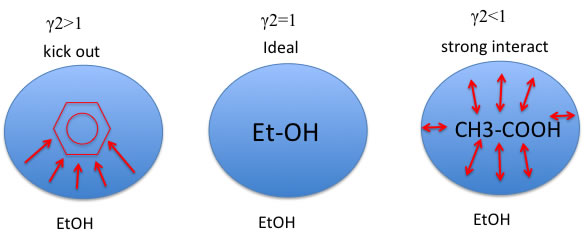This γis concentration dependent parameter. For example, Hexane-Acetone system, it become following chart.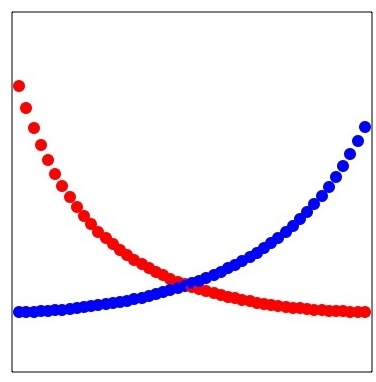At dilute condition, one molecule is surrounded by many other molecule, the force of putting out is increase. The value extrapolate to 0 is called infinite dilution activity coefficient.
If we have antoine parameters for individual molecule, and if we can estimate activity coefficient, we can calculate VLE for multi component system

ASOG method

The activity coefficient γi of component i in solution can be written with this scheme.

ln(γi) = ln(γiFH) + ln(γiG)

ln(γiFH): the size difference term
ln(γiG): group interaction term

ln(γiFH) is the function of heavy atom number.
For CH3:CH2:CH:C are determined 1:1:0.8:0.7.

ln(γiG) is determined each combination of functional groups that exist in system. Temperature affect this effect so that coefficients are also determined.

Journal of Chemical Engineering of Japan, functional groups pair are listed like below.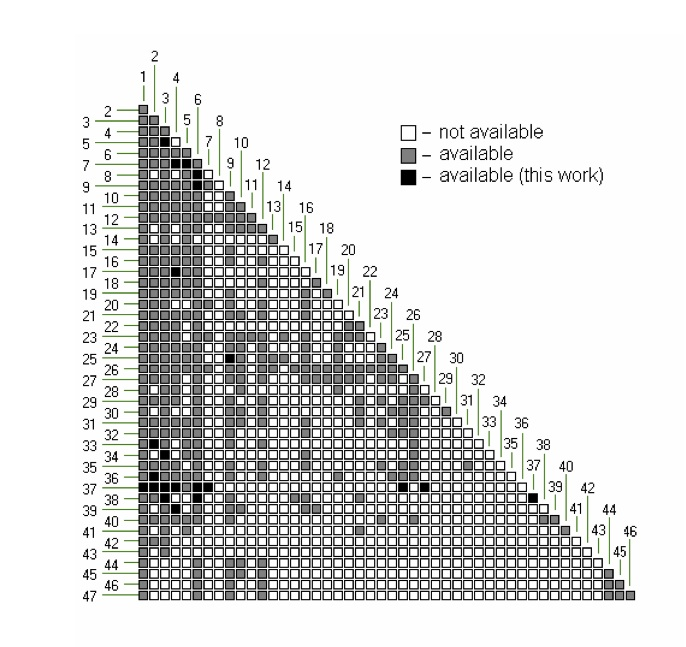I made sample program that can handle CH3(CH2,CH,C), OH, O=C<, COO, COOH only.